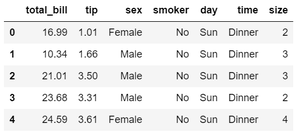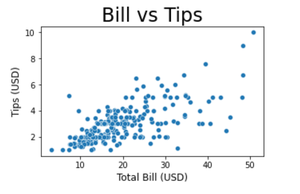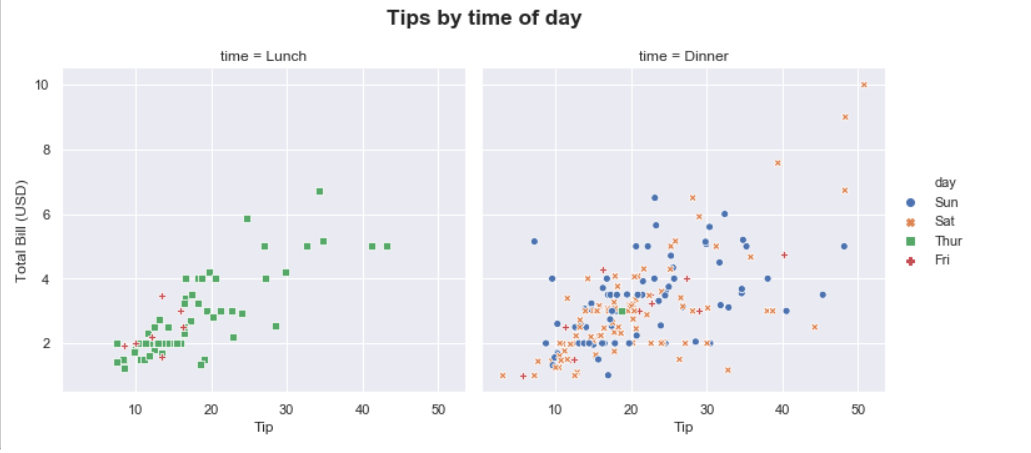# Change Axis Labels, Set Title and Figure Size to Plots with Seaborn

• Last Updated : 26 Nov, 2020

Seaborn is Python’s visualization library built as an extension to Matplotlib. Seaborn has Axes-level functions (scatterplot, regplot, boxplot, kdeplot, etc.) as well as Figure-level functions (lmplot, factorplot, jointplot, relplot etc.). Axes-level functions return Matplotlib axes objects with the plot drawn on them while figure-level functions include axes that are always organized in a meaningful way. The basic customization that a graph needs to make it understandable is setting the title, setting the axis labels, and adjusting the figure size. Any customization made is on the axes object for axes-level functions and the figure object for figure-level functions.

Note: Axes in the above explanation refers to a part of the figure or the top layer of a figure and is not the mathematical term for more than one axis. Consider a plot on a figure. This plot axes. Now, consider multiple subplots on a figure. Each of these subplots is one axes.

Let us see some examples to better understand customization with Seaborn.

## Python

 `# Import required libraries``import` `matplotlib.pyplot as plt``import` `seaborn as sns`` ` `# Load data set``tips ``=` `sns.load_dataset( ``"tips"` `)``tips.head()`

Output:Example 1: Customizing plot with axes object

For axes-level functions, pass the figsize argument to the plt.subplots() function to set the figure size. The function plt.subplots() returns Figure and Axes objects. These objects are created ahead of time and later the plots are drawn on it. We make use of the set_title(), set_xlabel(), and set_ylabel() functions to change axis labels and set the title for a plot. We can set the size of the text with size attribute. Make sure to assign the axes-level object while creating the plot. This object is then used for setting the title and labels as shown below.

## Python

 `# Set figure size (width, height) in inches``fig, ax ``=` `plt.subplots(figsize ``=` `( ``5` `, ``3` `))`` ` `# Plot the scatterplot``sns.scatterplot( ax ``=` `ax , x ``=` `"total_bill"` `, y ``=` `"tip"` `, data ``=` `tips )`` ` `# Set label for x-axis``ax.set_xlabel( ``"Total Bill (USD)"` `, size ``=` `12` `)`` ` `# Set label for y-axis``ax.set_ylabel( ``"Tips (USD)"` `, size ``=` `12` `)`` ` `# Set title for plot``ax.set_title( ``"Bill vs Tips"` `, size ``=` `24` `)`` ` `# Display figure``plt.show()`

Output:Example 2: Customizing scatter plot with pyplot object

We can also change the axis labels and set the plot title with the matplotlib.pyplot object using xlabel(), ylabel() and title() functions. Similar to the above example, we can set the size of the text with the size attribute. The function plt.figure() creates a Figure instance and the figsize argument allows to set the figure size.

## Python

 `# Set figure size (width, height) in inches``plt.figure(figsize ``=` `( ``5` `, ``3` `))`` ` `# Plot scatterplot``sns.scatterplot( x ``=` `"total_bill"` `, y ``=` `"tip"` `, data ``=` `tips )`` ` `# Set label for x-axis``plt.xlabel( ``"Total Bill (USD)"` `, size ``=` `12` `)`` ` `# Set label for y-axis``plt.ylabel( ``"Tips (USD)"` `, size ``=` `12` `)`` ` `# Set title for figure``plt.title( ``"Bill vs Tips"` `, size ``=` `24` `)`` ` `# Display figure``plt.show()`

Output:Example 3: Customizing multiple plots in the same figure

Seaborn’s relplot function returns a FacetGrid object which is a figure-level object. This object allows the convenient management of subplots. To give a title to the complete figure containing multiple subplots, we use the suptitle() method. The subplots_adjust() method is used to avoid overlapping of subplot titles and the figure title by specifying the top, bottom, left, and right edge positions of the subplots. To set the figure size, pass a dictionary with the key ‘figure.figsize’ in the set() method. The set() method allows to set multiple theme parameters in a single step.

## Python

 `# Set figure size``sns.``set``( rc ``=` `{``'figure.figsize'` `: ( ``20``, ``20` `), ``               ``'axes.labelsize'` `: ``12` `})`` ` `# Plot scatter plot``g ``=` `sns.relplot(data ``=` `tips , x ``=` `"total_bill"` `, ``                ``y ``=` `"tip"` `, col ``=` `"time"` `, ``                ``hue ``=` `"day"` `, style ``=` `"day"` `, ``                ``kind ``=` `"scatter"` `)`` ` `# Title for the complete figure``g.fig.suptitle(``"Tips by time of day"` `,``               ``fontsize ``=` `'x-large'` `, ``               ``fontweight ``=` `'bold'` `)`` ` `# Adjust subplots so that titles don't overlap``g.fig.subplots_adjust( top ``=` `0.85` `)`` ` `# Set x-axis and y-axis labels``g.set_axis_labels( ``"Tip"` `, ``"Total Bill (USD)"` `)`` ` `# Display the figure``plt.show()`

Output:My Personal Notes arrow_drop_up